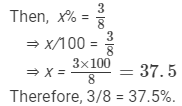Courses

# RD Sharma Solutions - Ex-11.5, Percentage, Class 7, Math Class 7 Notes | EduRev

## Class 7 : RD Sharma Solutions - Ex-11.5, Percentage, Class 7, Math Class 7 Notes | EduRev

The document RD Sharma Solutions - Ex-11.5, Percentage, Class 7, Math Class 7 Notes | EduRev is a part of the Class 7 Course RD Sharma Solutions for Class 7 Mathematics.
All you need of Class 7 at this link: Class 7

#### Question 1:

What per cent of
(i) 24 is 6?
(ii) Rs 125 is Rs 10?
(iii) 4 km is 160 metres?
(iv) Rs 8 is 25 paise
(v) 2 days is 8 hours?
(vi) 1 litre is 175 ml?

Let the number be x.
(i) 24% of x = 6
⇒ (24/100) × x = 6
⇒ x = 600/24 = 25%

(ii) 125% of   = 10
⇒ (125/100) ×  x = 10
⇒ x = 1000/125 = 8%

(iii) 4 km = 4000 metres (1 km = 1000 m)
4000% of  x = 160
⇒ (4000/100) × x =  160
⇒  x = 16000/4000  =  4%

(iv) Rs. 8 = 800 paise (Re. 1 = 100 paise)
800%  of x =  25
⇒ (800/ 100) × x = 25
⇒  x = 2500/800 = 25/8 = 3.125%

(v) 2 days = 2 × 24 = 48 (1 day = 24 hours)
48% of x = 8
⇒ (48/100)  ×  x =  8
⇒  x = 800/48 = 100/6 = 16.66%

(vi) 1 litre = 1000 ml
1000% of x = 175
⇒ (1000/100)  × x = 175
⇒ x = 17500/1000 = 175/10 = 17.5%

#### Question 2:

What per cent is equivalent to 3/8?

Let the percentage equivalent to 3/8 be x.#### Question 3:

Find the following:
(i) 8 is 4% of which number?
(ii) 6 is 60% of which number?
(iii) 6 is 30% of which number?
(iv) 12 is 25% of which number?

Let the number be x.

(i) 4% of  = 8
⇒ (4/100) × x = 8
⇒ x = 800/4 = 200

(ii) 60% of x = 6
⇒  (60/100) ×  = 6
⇒   x = 600/60 = 10

(iii) 30% of x = 6
⇒ (30/100) × x = 6
⇒ x = 600/30 = 20

(iv) 25% of  x = 12
⇒ (25/100) × x = 12
⇒   x = 1200/25 = 48

#### Question 4:

Convert each of the following pairs into percentages and find out which is more?
(i) 25 marks out of 30, 35 marks out of 40.
(ii) 100 runs scored off 110 balls, 50 runs scored off 55 balls.

(i) 25 marks out of 30
⇒ (25/30) × 100 = 2500/30 = 83.33%
35 marks out of  40
⇒ (35/40) × 100 = 3500/40 = 87.5%
87.5% is greater than 83.33%.

(ii) 100 runs scored off 110 balls
⇒ (100/110) × 100 = 10000/110 = 90.90%
50  runs scored off  55 balls
⇒ (50/55) × 100 = 5000/55 = 90.90%
Both percentages are equal.

#### Question 5:

Find 20% more than Rs 200.

20% more than 200 means 20% of 200 plus 200.
That is, {(20/100) × 200} + 200 = (4000/100) + 200 = 40 + 200 = 240.

#### Question 6:

Find 10% less than Rs 150.

10% less than Rs. 150 means 150 minus 10% of 150.
That is, 150 - {(10/100) × 150}  = 150 - (1500/100) = 150 - 15  = Rs. 135.

Offer running on EduRev: Apply code STAYHOME200 to get INR 200 off on our premium plan EduRev Infinity!

97 docs

,

,

,

,

,

,

,

,

,

,

,

,

,

,

,

,

,

,

,

,

,

,

,

,

,

,

,

,

,

,

;